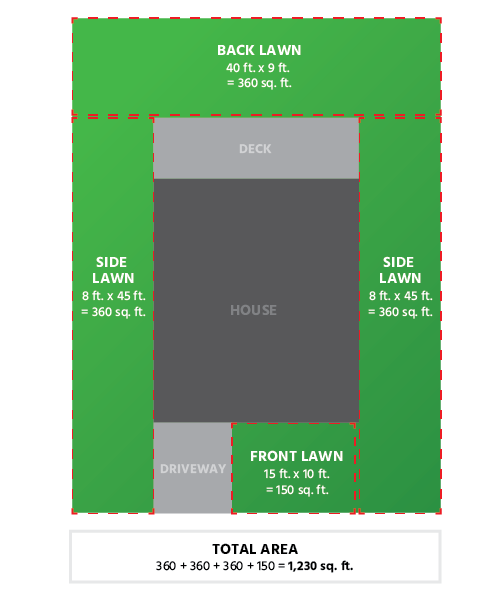## Help! I don’t know where to start!

Don’t worry – Sodmasters has your back. Estimating how much sod your space requires might seem like a daunting task, especially if the shape of the space is complex. Fortunately, for even the most oblong yards, measurement and estimation can be made simple and manageable. In all cases, your goal will be to calculate the total area of the space in square feet.

## Measuring a rectangular space

To measure a rectangular space for sod installation, simply take the length of the space and multiply it by the width to determine the total area.

For example, if you are measuring a rectangular lawn which is 30 feet wide and 10 feet long, the total area is 300 square feet.## Measuring a more complex space

For unconventionally shaped spaces, measuring the area is almost as easy as measuring a rectangular one. Begin by dividing your space into smaller, rectangular boxes and take the area of each box. Then, add all of the areas together for a total measurement of the amount of sod you will need.

An easy way to divide your space into separate rectangular spaces is to carefully draw the shape of the space onto a sheet of graph paper. After sketching the outline, identify all of the areas which can be contained by a single rectangular shape and draw rectangles around those areas, preferably in a different color. Next, simply measure the areas of each rectangle (multiply the width and length to determine the total area of each shape). Finally, add all of the areas together to derive the total area of the space.## Tips

• When measuring your space, don’t forget to subtract the area of any spots that won’t need sod.
• If your lawn is uneven or bumpy, or if you’re just feeling unsure about your measurements, consider increasing your estimate by 10%.
• While a tape measure is sufficient for measuring the lawns of most homes, when measuring larger spaces, consider using a dedicated measuring wheel for  convenience and accuracy.